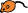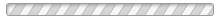Bionumbers Browser    [?] ×Browse all available Bionumbers:

or select:

Organism:
Type of Bionumber (by units):
Bionumber:

or search:

Enter what you want to calculate, using the # symbol to denote any uncertain quantities, e.g. 4/3*pi*#cellRadius^3. Caladis will then ask you for information about uncertain quantities, and calculate the probabilities of different answers.
Go ahead! Use these buttons and/or the space above to input an expression to calculate.

Calculate
• Standard Deviation Analysis [?]:

• Sample size [?]:

• Binning method [?]:

• Angle unit [?]:

• Interpret Bionumbers [?]: